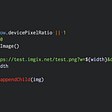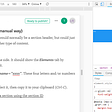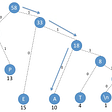# Longest Consecutive Sequence — Day 29(Python)

Today I stumbled upon an interesting problem. I thought it would be great to share the solution in Medium. It is one of the Hard tagged questions in Leetcode.

128. Longest Consecutive Sequence

Given an unsorted array of integers `nums`, return the length of the longest consecutive elements sequence.

Follow up: Could you implement the `O(n)` solution?

Example 1:

`Input: nums = [100,4,200,1,3,2]Output: 4Explanation: The longest consecutive elements sequence is [1, 2, 3, 4]. Therefore its length is 4.`

Example 2:

`Input: nums = [0,3,7,2,5,8,4,6,0,1]Output: 9`

Constraints:

• `0 <= nums.length <= 104`
• `-109 <= nums[i] <= 109`

The first thought that came into my mind was to sort the list and check for consecutive numbers. Let us look into the algorithm.

1. Sort the array.
2. Let us have a counter that keeps track of numbers in the current consecutive sequence. Let us have another counter that keeps track of max among all the counter values.
3. Pop the first value from the list and increment the counter value.
4. Now, start a loop until elements are present in the array.
5. Check if the first number in the list is consecutive to the earlier number. If yes, increment the counter. If both the number is equal, then remove it from the list. If the new number is not consecutive, restart the counter from 1.
6. Check if the current counter is higher than the earlier counter.
7. Pop the first number from the array and go through the loop.

The code snippet looks like below.

`class LongestConsecutiveFinder:    def longestConsecutive(self, nums: List[int]) -> int:        if len(nums) == 0:            return 0        nums = sorted(nums)        count = 0        max_count = 0        c_num = nums.pop(0)        count += 1        max_count = max(count, max_count)        while(nums):            if c_num + 1 == nums:                count += 1            elif c_num == nums:                nums.pop(0)                continue            else:                count = 1            max_count = max(count, max_count)            c_num = nums.pop(0)        return(max_count)`

Complexity analysis.

Time Complexity

We are sorting the list before running the algorithm, which takes O(NlogN). While using the algorithm, we are just traversing through the entire list which takes O(N). Hence the time taken is O(NlogN).

Space Complexity

We are not using any extra data structure to store any intermediate results, and hence the space complexity is O(1).

Can we try to improve the solution? Can we solve the problem in O(N)?

1. We need to convert the given list into a set to ensure we can access elements in O(1) time.
2. Take each number from the set, check if its predecessor is present in the set. If yes, continue with the loop; else, go to the next step. The idea behind this step is to get the start of the streak.
3. Check if the successor of the given number is present in the set. If yes, keep checking until we find the end of the consecutive streak.
4. We have to keep track of the length of the streak and find the maximum among the streaks that we have.
`class LongestConsecutiveFinder:    def longestConsecutive(self, nums: List[int]) -> int:        nums = set(nums)        count = 0        for x in nums:            if x-1 not in nums:                y = x + 1                while y in nums:                    y += 1                count = max(count, y-x)        return count`

Complexity analysis.

Time Complexity

We are converting the list into a set and traversing through the set. Therefore the time complexity is O(N).

Space Complexity

We are not using any extra data structure to store any intermediate results, and hence the space complexity is O(1).

I have used the logic for this code from Stefan Pochmann.

I would love to hear your feedback about my posts. Do let me know if you have any comments or feedback.

--

--

--

## More from Annamariya Tharayil

Software Engineer. Find me @ www.linkedin.com/in/annamariya-jt

Love podcasts or audiobooks? Learn on the go with our new app.

## How imgix Changed the Way I Build Apps## Simple AWS SQS queue producer and consumer## Just DO IT!## AOA Bi-Monthly Technology Update| April-May 2022## Parsing CSVs with Modern C++## Why Spectra?## Annamariya Tharayil

Software Engineer. Find me @ www.linkedin.com/in/annamariya-jt

## Introduction to Programming - What is Programming Paradigm?## Remove Nth Node From End of List — Day 107(Python)## Huffman coding | Greedy Algorithm## Memory Management : free()